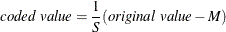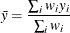Displayed Output

All estimates and hypothesis tests assume that the model is correctly specified and the errors are distributed according to classical statistical assumptions.

The output displayed by PROC RSREG includes the following.

Estimation and Analysis of Variance

• The actual form of the coding operation for each value of a variable iswhere M is the average of the highest and lowest values for the variable in the design and S is half their difference. The Subtracted off column contains the M values for this formula for each factor variable, and S is found in the Divided by column.

• The summary table for the response variable contains the following information.

• Response Mean is the mean of the response variable in the sample. When a WEIGHT statement is specified, the meanis calculated by• Root MSE estimates the standard deviation of the response variable and is calculated as the square root of the Total Error mean square.

• The R-Square value is, or the coefficient of determination.measures the proportion of the variation in the response that is attributed to the model rather than to random error.

• The Coefficient of Variation is 100 times the ratio of the Root MSE to the Response Mean.

• A table analyzing the significance of the terms of the regression is displayed. Terms are brought into the regression in four steps: (1) the Intercept and any covariates in the model, (2) Linear terms like X1 and X2, (3) pure Quadratic terms like X1*X1 or X2*X2, and (4) Crossproduct terms like X1*X2. The table displays the following information:

• the degrees of freedom in the DF column, which should be the same as the number of corresponding parameters unless one or more of the parameters are not estimable

• Type I Sum of Squares, also called the sequential sums of squares, which measures the reduction in the error sum of squares as sets of terms (Linear, Quadratic, and so forth) are added to the model

• R-Square, which measures the portion of totalcontributed as each set of terms (Linear, Quadratic, and so forth) is added to the model

• F Value, which tests the null hypothesis that all parameters in the term are zero by using the Total Error mean square as the denominator. This is a test of a Type I hypothesis, containing the usual F test numerator, conditional on the effects of subsequent variables not being in the model.

• Pr > F, which is the significance value or probability of obtaining at least as great an F ratio given that the null hypothesis is true.

• The Sum of Squares column partitions the Total Error into Lack of Fit and Pure Error. When Lack of Fit is significant, there is variation around the model other than random error (such as cubic effects of the factor variables).

• The Total Error Mean Square estimates, the variance.

• F Value tests the null hypothesis that the variation is adequately described by random error.

• A table containing the parameter estimates from the model is displayed.

• The Estimate column contains the parameter estimates based on the uncoded values of the factor variables. If an effect is a linear combination of previous effects, the parameter for the effect is not estimable. When this happens, the degrees of freedom are zero, the parameter estimate is set to zero, and estimates and tests on other parameters are conditional on this parameter being zero.

• The Standard Error column contains the estimated standard deviations of the parameter estimates based on uncoded data.

• The t Value column contains t values of a test of the null hypothesis that the true parameter is zero when the uncoded values of the factor variables are used.

• The Pr > |T| column gives the significance value or probability of a greater absolute t ratio given that the true parameter is zero.

• The Parameter Estimate from Coded Data column contains the parameter estimates based on the coded values of the factor variables. These are the estimates used in the subsequent canonical and ridge analyses.

• The sum of squares are partitioned by the factors in the model, and an analysis table is displayed. The test on a factor is a joint test on all the parameters involving that factor. For example, the test for the factor X1 tests the null hypothesis that the true parameters for X1, X1*X1, and X1*X2 are all zero.

Canonical Analysis

• The Critical Value columns contain the values of the factor variables that correspond to the stationary point of the fitted response surface. The critical values can be at a minimum, maximum, or saddle point.

• The eigenvalues and eigenvectors are from the matrix of quadratic parameter estimates based on the coded data. They characterize the shape of the response surface.

Ridge Analysis

• The Coded Radius column contains the distance from the coded version of the associated point to the coded version of the origin of the ridge. The origin is given by the point at radius zero.

• The Estimated Response column contains the estimated value of the response variable at the associated point. The standard error of this estimate is also given. This quantity is useful for assessing the relative credibility of the prediction at a given radius. Typically, this standard error increases rapidly as the ridge moves up to and beyond the design perimeter, reflecting the inherent difficulty of making predictions beyond the range of experimentation.

• The Uncoded Factor Values columns contain the values of the uncoded factor variables that give the optimum response at this radius from the ridge origin.